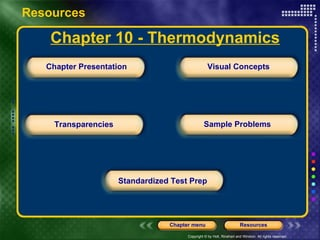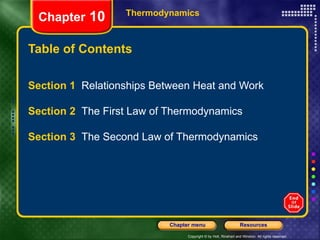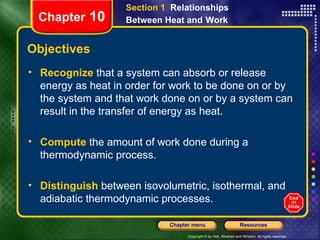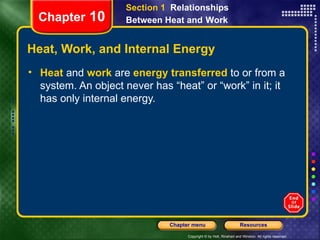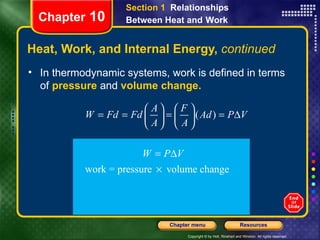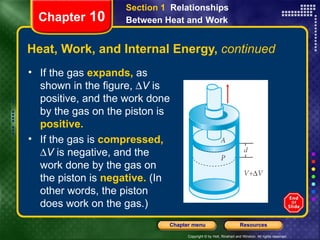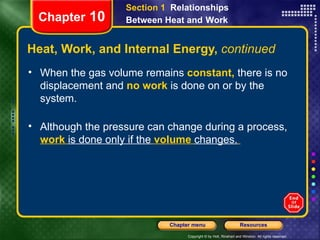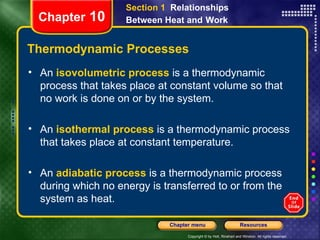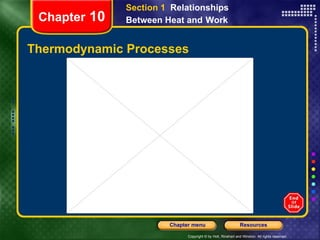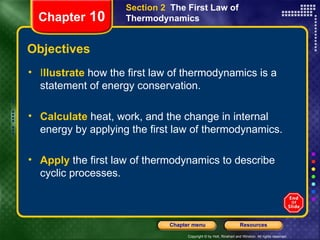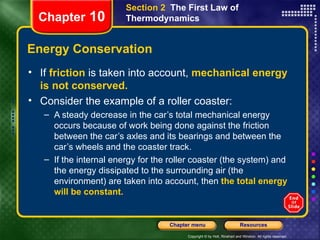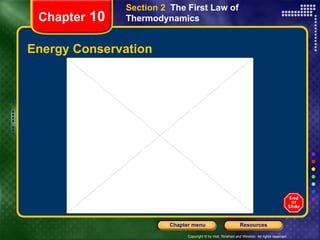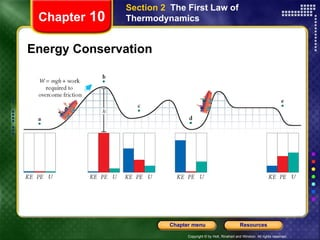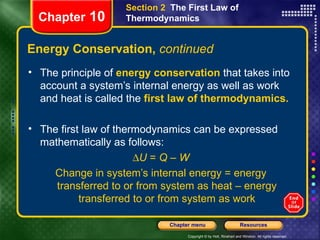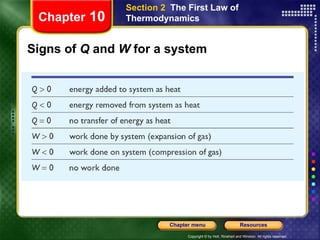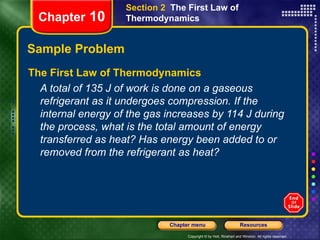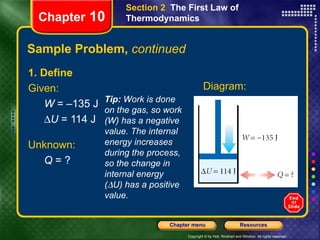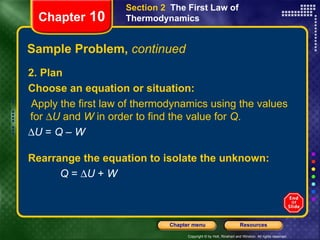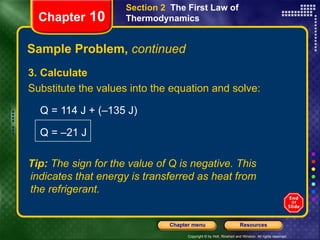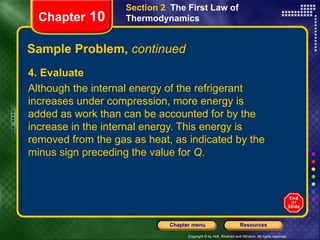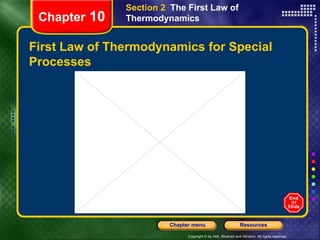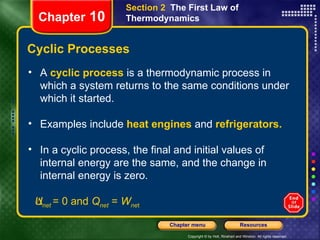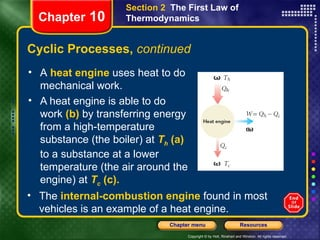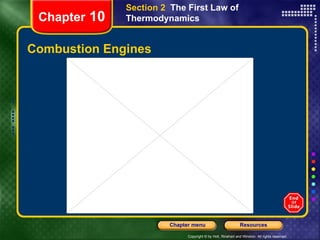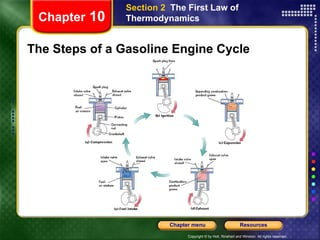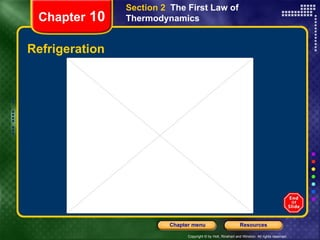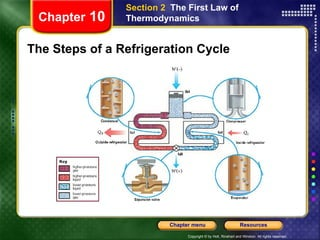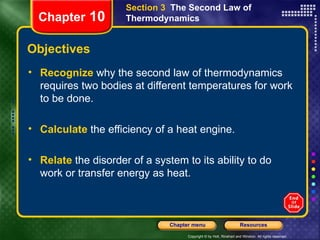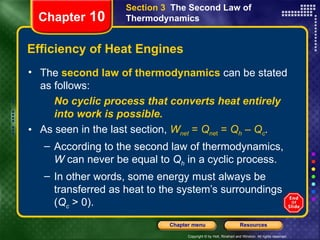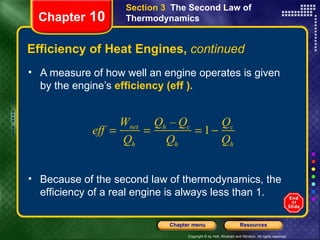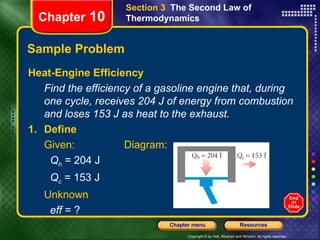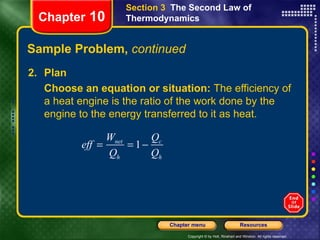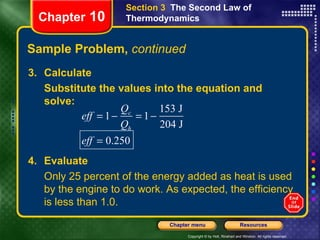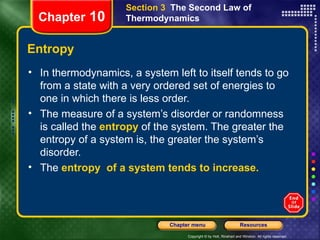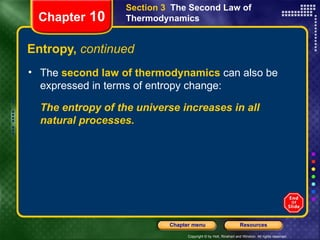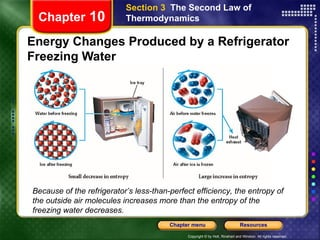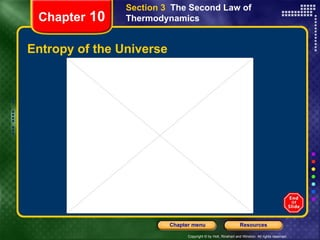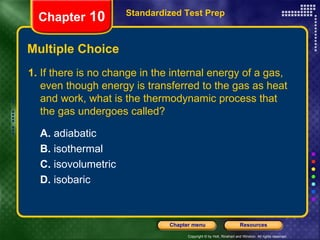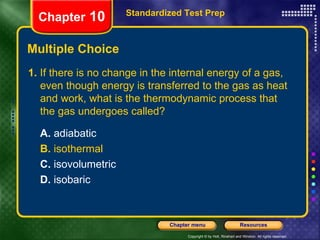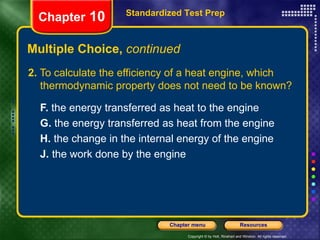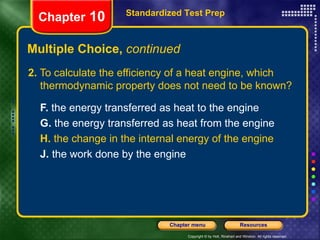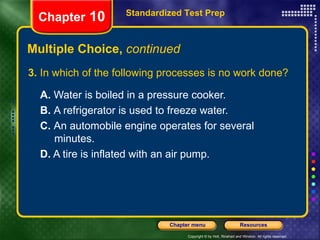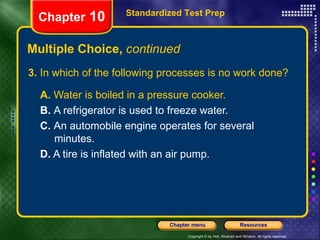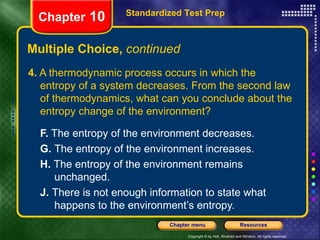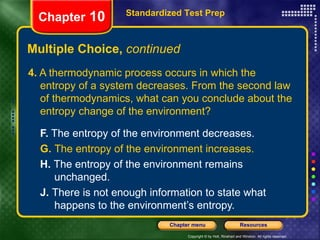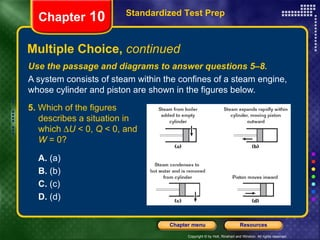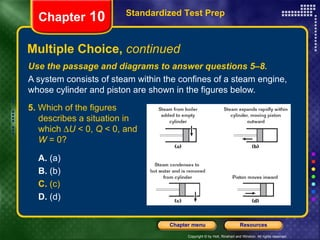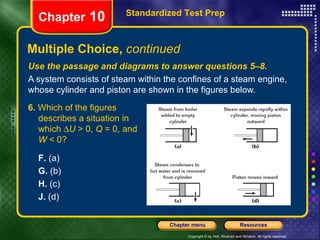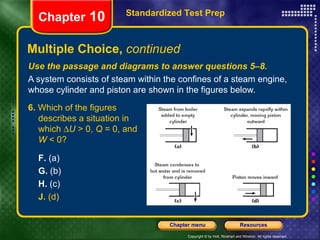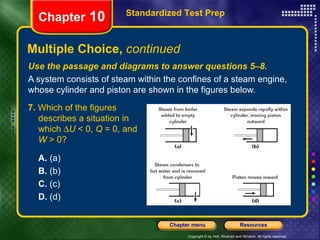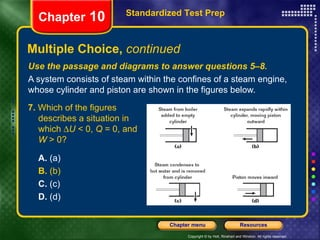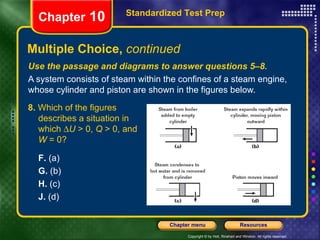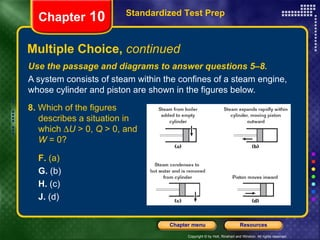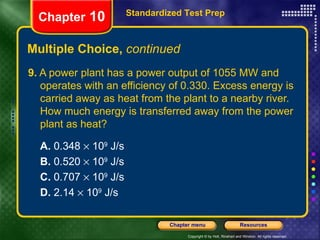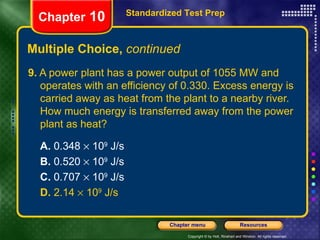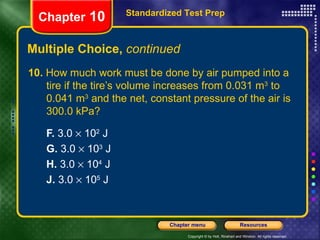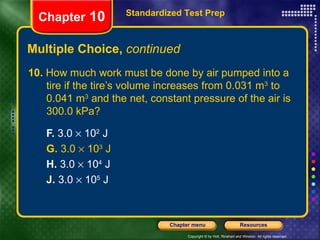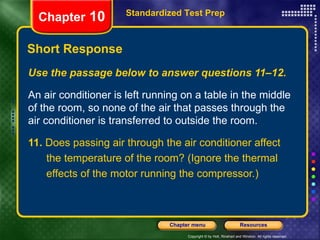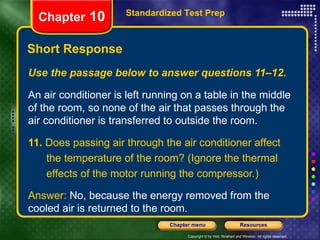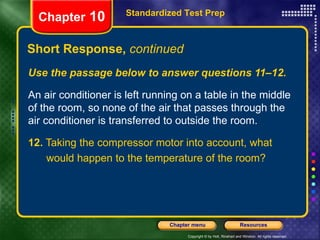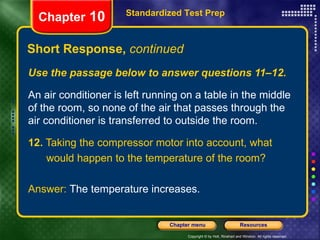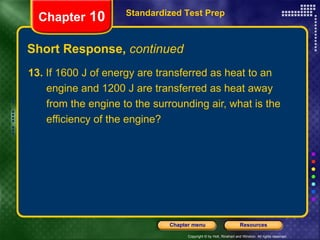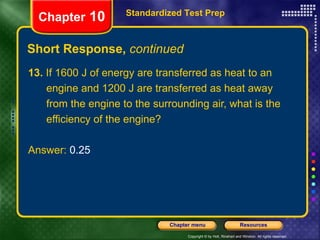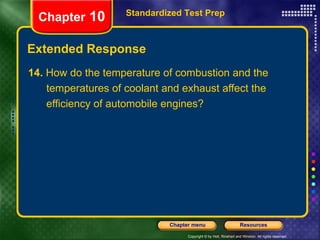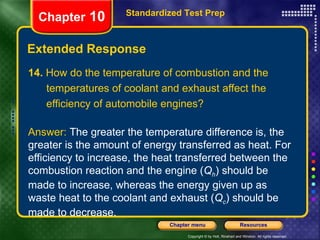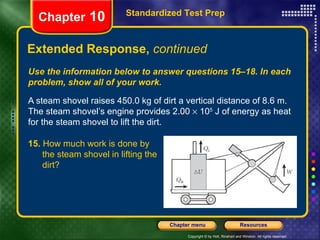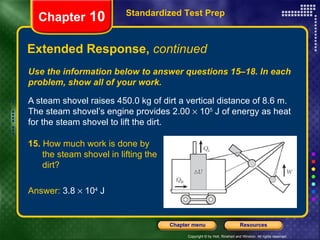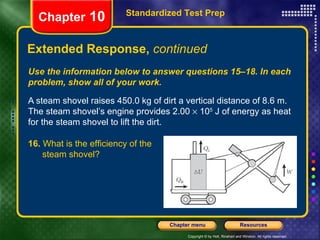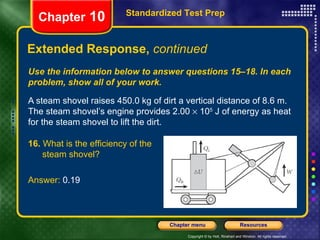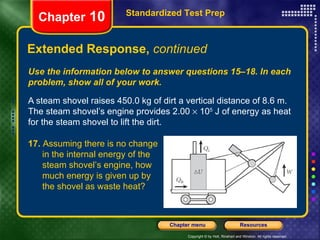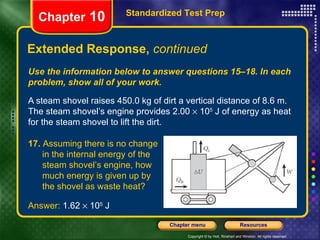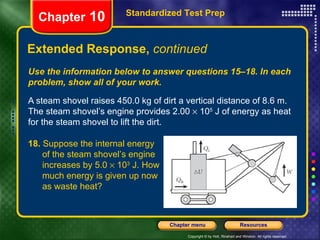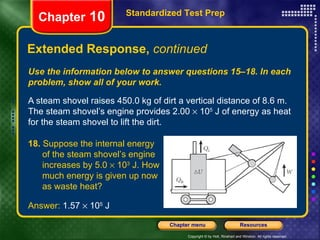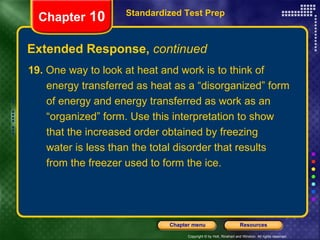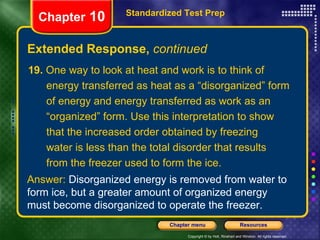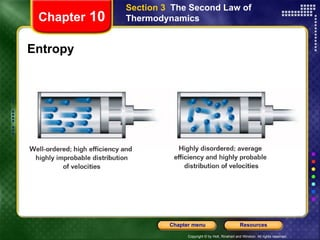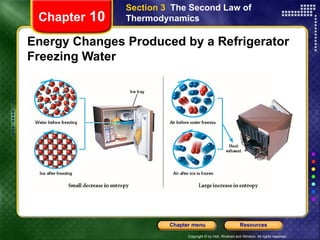1 of 77

### Chapter 10 Powerpoint

1. Chapter Presentation Transparencies Sample Problems Visual Concepts Standardized Test Prep Resources Chapter 10 - Thermodynamics
2. Thermodynamic Processes Chapter 10 Section 1 Relationships Between Heat and Work
3. Energy Conservation Chapter 10 Section 2 The First Law of Thermodynamics
4. Energy Conservation Chapter 10 Section 2 The First Law of Thermodynamics
5. Signs of Q and W for a system Chapter 10 Section 2 The First Law of Thermodynamics
6. First Law of Thermodynamics for Special Processes Chapter 10 Section 2 The First Law of Thermodynamics
7. Combustion Engines Chapter 10 Section 2 The First Law of Thermodynamics
8. The Steps of a Gasoline Engine Cycle Chapter 10 Section 2 The First Law of Thermodynamics
9. Refrigeration Chapter 10 Section 2 The First Law of Thermodynamics
10. The Steps of a Refrigeration Cycle Chapter 10 Section 2 The First Law of Thermodynamics
11. Energy Changes Produced by a Refrigerator Freezing Water Chapter 10 Section 3 The Second Law of Thermodynamics Because of the refrigerator’s less-than-perfect efficiency, the entropy of the outside air molecules increases more than the entropy of the freezing water decreases.
12. Entropy of the Universe Chapter 10 Section 3 The Second Law of Thermodynamics
13. Entropy Chapter 10 Section 3 The Second Law of Thermodynamics
14. Energy Changes Produced by a Refrigerator Freezing Water Chapter 10 Section 3 The Second Law of Thermodynamics